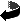# On the geometry of Riemannian manifolds with a Lie structure at infinity by Bernd Ammann, Robert Lauter, Victor Nistor

On the geometry of Riemannian manifolds with a Lie structure at infinity (.dvi, .ps,.ps.gz oder .pdf)
Int. J. Math. and Math. Sciences 2004:4 (2004) 161--193,
DOI 10.1155/S0161171204212108

### Abstract

A manifold with a ``Lie structure at infinity'' is a non-compact manifold \$M_0\$ whose geometry is described by a compactification to a manifold with corners \$M\$ and a Lie algebra of vector fields on \$M\$ subject to constraints only on \$M \smallsetminus M_0\$. The Lie structure at infinity on \$M_0\$ determines a metric on \$M_0\$ up to bi-Lipschitz equivalence. This leads to the natural problem of understanding the Riemannian geometry of these manifolds, which is the main question addressed in this paper.
We prove, for example, that on a manifold with a Lie structure at infinity the curvature tensor and its covariant derivatives are bounded. We also study a generalization of the geodesic spray and give conditions for these manifolds to have positive injectivity radius.
An important motivation for our study is to prepare the ground for the investigation of the analysis of geometric operators on manifolds with a Lie structure at infinity. The simplest examples of manifolds with a Lie structure at infinity are the manifolds with cylindrical ends. For these manifolds the relevant analysis is that of totally characteristic operators on a compact manifold with boundary equipped with a ``\$b\$-metric.''
Our program can thus be thought of as a generalization of the known results on totally characteristic operators.

### Keywords

Non-compact manifolds, geometry at infinity, Lie structures, asymptotically flat, asymptotically hyperbolicZurück zur Homepage

The Paper was written on 10.1.2002
Last update 12.3.2004